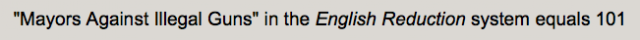## Thursday, June 12, 2014

### 74 Shootings Since Sandy Hook Stat Origin | Michael Bloomberg's "Everytown for Gun Safety"

I had been wondering where the comical stat came from in regards to the shooting in Troutdale, Oregon being the "74 shooting" since Sandy Hook on December 14, 2012.
• Oregon is the only state with 74 numerology
• Oregon = 15+18+5+7+15+14 = 74
• Oregon is the 33rd State
• OR = 15+18 = 33
• Jesus was crucified at 33
• Jesus = 10+5+19+21+19 = 74
• Cross = 3+18+15+19+19 = 74

Thanks to CNN, that is no longer a question of mine.  The stat came from Michael Bloomberg's start-up, 'Everytown for Gun Safety'.
• Michael = 4+9+3+8+1+5+3 = 33
• Bloomberg = 2+3+6+6+4+2+5+9+7 = 44
• Michael Bloomberg = 33+44 = 77
"Everytown for Gun Safety"... what a name.  How about instead, "Everytown for legit news".
• Everytown = 5+22+5+9+7+2+6+5+5 = 66
• for = 6+6+9 = 21
• Gun = 7+3+5 = 15
• Safety = 1+1+6+5+2+7 = 22
With regards to the word "gun", it can also be reduced with the Simple Method.
• Gun = 7+21+14 = 42
In this regard, it has special numerology, and matches that of sin and war in terms of three letter words that have a value of 42.
• Sin = 19+9+14 = 42
• War = 23+1+18 = 42

Notice that because of Thomas Menino's October 30, 2014 death, Michael Bloomberg is the head of this lobby for changing gun laws, now called Everytown for Gun Safety, once called Mayors Against Illegal Guns.Assassin = 101

The date Thomas Menino died on has very familiar numerology.

October 30, 2014

10/30/2014 = 10+30+20+14 = 74 (Masonic = 74)
10/30/2014 = 10+30+(2+0+1+4) = 47 (Obey, authority, judge, gavel, mafia)
10/30/2014 = 1+0+3+0+2+0+1+4 = 11
10/30/14 = 10+30+14 = 54

October is the only month with '33' Gematria.  We know how these Masons love to pair 33 and 74.

October = 6+3+2+6+2+5+9 = 33

Notice he died from cancer, at least supposedly.

Cancer = 3+1+14+3+5+18 = 44
Chemo = 3+8+5+13+15 = 44
Kill = 11+9+12+12 = 44

http://www.ijreview.com/2014/06/146537-everytown-map-74-school-shootings-since-newtown-goes-viral-33-questionable-entries/

Notice the claim that 1.37 school shootings have occurred every week since Newtown, December 14, 2015.  Keep in mind that 137 is the 33rd prime number, and a number that is often coded in these hoaxes.